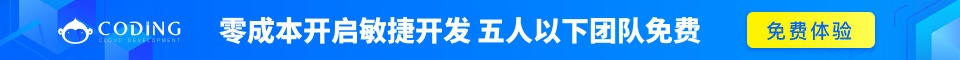# Ruby 判断

Ruby 提供了其他现代语言中很常见的条件结构。在这里，我们将解释所有的条件语句和 Ruby 中可用的修饰符。

## 语法

```if conditional [then]
code...
[elsif conditional [then]
code...]...
[else
code...]
end
```

if 表达式用于条件执行。值 falsenil 为假，其他值都为真。请注意，Ruby 使用 elsif，不是使用 else if 和 elif。

if 表达式的 conditional 通过保留字 then、一个换行符或一个分号，来与代码分离开。

## 实例

```#!/usr/bin/ruby

x=1
if x > 2
puts "x is greater than 2"
elsif x <= 2 and x!=0
puts "x is 1"
else
puts "I can't guess the number"
end
```
```x is 1
```

## 语法

```code if condition
```

## 实例

```#!/usr/bin/ruby

\$debug=1
print "debug\n" if \$debug
```

```debug
```

## 语法

```unless conditional [then]
code
[else
code ]
end
```

## 实例

```#!/usr/bin/ruby

x=1
unless x>2
puts "x is less than 2"
else
puts "x is greater than 2"
end
```

```x is less than 2
```

## 语法

```code unless conditional
```

## 实例

```#!/usr/bin/ruby

\$var =  1
print "1 -- Value is set\n" if \$var
print "2 -- Value is set\n" unless \$var

\$var = false
print "3 -- Value is set\n" unless \$var
```

```1 -- Value is set
3 -- Value is set
```

## 语法

```case expression
[when expression [, expression ...] [then]
code ]...
[else
code ]
end
```

when 子句所指定的 expression 背当作左操作数。如果没有匹配的 when 子句，case 执行 else 子句的代码。

when 语句的表达式通过保留字 then、一个换行符或一个分号，来与代码分离开。

```case expr0
when expr1, expr2
stmt1
when expr3, expr4
stmt2
else
stmt3
end
```

```_tmp = expr0
if expr1 === _tmp || expr2 === _tmp
stmt1
elsif expr3 === _tmp || expr4 === _tmp
stmt2
else
stmt3
end
```

## 实例

```#!/usr/bin/ruby

\$age =  5
case \$age
when 0 .. 2
puts "baby"
when 3 .. 6
puts "little child"
when 7 .. 12
puts "child"
when 13 .. 18
puts "youth"
else
```little child